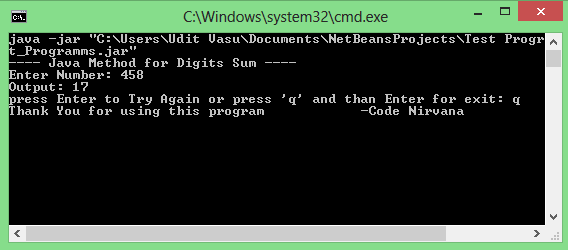# Java Method: Sum of all the digits of a number

How to create a Java program which adds all the given digits of a number ?

If you are looking for this..... you came to the right place.
The above question can be solved easily by using the give Java Method on Digit Sum.

//METHOD FOR Digits SUM
```    int addDigits (int n)
{
int sum=0;
int dig;
while(n>0){
dig = n%10;
sum=sum+dig;
n= n/10;
}
return sum;
}
```
/*
*this will give following output: 1+0+1=2
*output will be: 9+9=18
*/

If you want to explore more on this.... Try the following Java Program using the above Digit Sum Method :
```import java.io.BufferedReader;
import java.io.IOException;

public class digitSum{
public static void main( String arg[]) throws IOException {
System.out.println("------------ Java Method for Digits Sum ------------");
for(int i=1;i>=0;i++){      //Used for try again condition
int num=0;
try{
System.out.print("Enter Number: ");
}catch ( Exception Number ){
System.out.println("******** Illegal Input! ********");
continue;
}
System.out.println("Output: "+num);
System.out.print("press Enter to Try Again or press 'q' and than Enter for exit: ");
if(opp.equalsIgnoreCase("q")){
System.out.println("Thank You for using this program            -Code Nirvana");
break;
}
}
}
public static int addDigits (int n)
{
int sum=0;
int dig;
while(n>0){
dig = n%10;
sum=sum+dig;
n= n/10;
}
return sum;
}
}
```

Output :